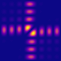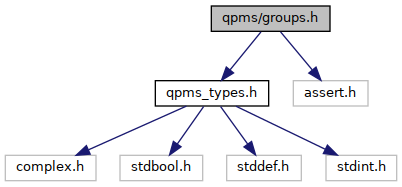QPMS Electromagnetic multiple scattering library and toolkit.
groups.h File Reference

Point groups. More...

#include "qpms_types.h"
#include <assert.h>
Include dependency graph for groups.h:Go to the source code of this file.

## Data Structures

struct  qpms_finite_group_irrep_t
To be used only in qpms_finite_group_t. More...

struct  qpms_finite_group_t
A point group with its irreducible representations and some metadata. More...

## Typedefs

typedef struct qpms_finite_group_t qpms_finite_group_t
A point group with its irreducible representations and some metadata. More...

## Functions

static qpms_gmi_t qpms_finite_group_mul (const qpms_finite_group_t *G, qpms_gmi_t a, qpms_gmi_t b)
Group multiplication.

static qpms_gmi_t qpms_finite_group_inv (const qpms_finite_group_t *G, qpms_gmi_t a)
Group element inversion.

static _Bool qpms_iri_is_valid (const qpms_finite_group_t *G, qpms_iri_t iri)

qpms_iri_t qpms_finite_group_find_irrep_by_name (qpms_finite_group_t *G, char *name)
NOT IMPLEMENTED Get irrep index by name.

## Variables

const qpms_finite_group_t QPMS_FINITE_GROUP_TRIVIAL
Trivial group.

const qpms_finite_group_t QPMS_FINITE_GROUP_TRIVIAL_G
Trivial group, with one (reduntant) generator. More...

## Detailed Description

Point groups.

Right now, the instances of qpms_finite_group_t are created at compilation time from source code generated by Python script TODO (output groups.c) and they are not to be constructed dynamically.

In the end, I might want to have a special type for 3D point groups or more specifically, for the closed subgroups of O(3), see https://en.wikipedia.org/wiki/Point_groups_in_three_dimensions. They consist of the seven infinite series of axial groups (characterized by the series index, the axis direction, and the index n of the n-fold rotational symmetry) and the seven remaining point groups + the finite groups. All off them have a quite limited number of generators (max. 4?; CHECKME). The goal is to have some representation that would enable to

1. fully describe the symmetries of abstract T-matrices/nanoparticles,
2. quickly determine e.g. whether one is a subgroup of another,
3. have all the irreps,
4. have all in C and without excessive external dependencies, etc.

## ◆ qpms_finite_group_t

 typedef struct qpms_finite_group_t qpms_finite_group_t

A point group with its irreducible representations and some metadata.

The structure of the group is given by the multiplication table mt.

Each element of the group has its index from 0 to order. The metadata about some element are then accessed using that index.

All members are in principle optional except order and mt.

Note: after changing this struct, don't forget to update the Python method SVWFPointGroupInfo.generate_c_source().

## ◆ QPMS_FINITE_GROUP_TRIVIAL_G

 const qpms_finite_group_t QPMS_FINITE_GROUP_TRIVIAL_G
extern

Trivial group, with one (reduntant) generator.

For the trivial group, zero generators are enough. However, some functions might be not robust enough and require a first generator to work properly.